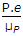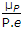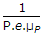# Electronics and Communication Engineering - Electronic Devices and Circuits

46.

Fermi level in intrinsic semiconductor is at the centre of forbidden energy band.

 A. True B. False

Explanation:

No answer description available for this question. Let us discuss.

47.

In a photo transistor the photocurrent is

 A. emitter base junction B. collector base junction C. collector D. either (a) or (b)

Explanation:

No answer description available for this question. Let us discuss.

48.

In photoelectric emission the maximum kinetic energy of emitted electron is proportional to

 A. f B. f C. f2 D. f3

Explanation:

No answer description available for this question. Let us discuss.

49.

In a P-type semiconductor, the conductivity due to holes (= σP) is equal to e = charge of hole, μP = hole mobility, P = hole concentration,

 A.B.C. P.e.μP D.Explanation:

No answer description available for this question. Let us discuss.

50.

Hall effect is observed in a specimen when it is carrying current and is placed in a magnetic field. The resulting electric field inside the speciment will be in

 A. direction normal to both current and magnetic field B. the direction of current C. direction antiparallel to magnetic field D. an arbitrary direction depend upon conductivity